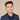# Get iOS Screen Size In Swift

To get the screen size in Swift, use the following code:

``````let screenSize: CGRect = UIScreen.main.bounds
let screenWidth = screenSize.width
let screenHeight = screenSize.height

print("The width is \(screenWidth) and the height is \(screenHeight)")
// For example, this will print:
// The width is 414.0 and the height is 896.0``````

## Get Safe Area Size

The safe area is the the space between the top inset and the bottom inset. In this photo, it is the green colored part:To get the size of the safe area use this code:

``````let window = UIApplication.shared.windows
let safeFrame = window.safeAreaLayoutGuide.layoutFrame
let width = safeFrame.width
let height = safeFrame.height
print("The safe area width is \(width) and the safe area height is \(height)")
// For example, this will print:
// The safe area width is 414.0 and the safe area height is 818.0``````

To get the size of the insets (the red part in the above image), use this following code:

``````let window = UIApplication.shared.windows